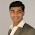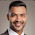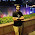## Index

 STA & SI Chapter1 Chapter2 Chapter3 Chapter4 Chapter5 Chapter6 Chapter7 Chapter8 Introduction Static Timing Analysis Clock Advance STA Signal Integrity EDA Tools Timing Models Other Topics

 Extraction & DFM Chapter1 Chapter2 Chapter3 Chapter4 Chapter5 Chapter6 Introduction Parasitic Interconnect Corner (RC Corner) Manufacturing Effects and Their Modeling Dielectric Layer Process Variation Other Topic

# How To Solve Setup and Hold Violation.. continue...

 STA & SI:: Chapter 2: Static Timing Analysis 2.1 2.2 2.3a 2.3b 2.3c 2.4a Timing Paths Time Borrowing Basic Concept Of Setup-Hold Basic Concept of Setup-Hold Violation Examples:S-H Time/Violation Timing Path Delay 2.4b 2.4c 2.5a 2.5b 2.6a 2.6b Interconnect Delay Models Delay - Wire Load Model Maximum Clock Frequency Calculate “Max Clock Freq”-Examples Fix Setup-Hold Violation-1 Fix Setup-Hold Violation-2 2.6c 2.7a 2.7b 2.7c 2.8 Fix Setup-Hold Violation-3 Incr/Decr Delay Method-1 Incr/Decr Delay Method-2 Incr/Decr Delay Method-3 10 ways to fix Setup-Hold Violation.

Static Timing analysis is divided into several parts:

In the last part/post we have discussed 2 more examples with different specifications with more restrictions (Both net delay and Tck2Q were ideal means 0ns) and figure out that if you want to fix the violation by increasing/decreasing the delay in the data path then following condition should be satisfied.

Min delay of Combinational path > Hold time of Capture FF.
Max delay of Combinational path < Clock Period - Setup time of Capture FF.

Clock Period >= Setup time + Hold time.

But in case if you can’t touch the data path and you have to increase/decrease the delay in the clock path (means between “Clk pin to Launch FF clock pin” Or between “Clk pin and capture FF clock pin”), then following conditions should satisfied.

Max Delay/ Setup Condition:
Td < Clk_Period + (T_capture - T_launch) – T_setup

Min Delay / Hold Condition:
Td >  (T_capture -  T_launch ) + T_hold

Where:
Td -> Combinational path delay (between the 2 FFs)
T_capture -> Delay of circuit present between “Clk pin and capture FF clock pin”
T_launch -> Delay of circuit present between “Clk pin to Launch FF clock pin”

In this post we will discuss few more examples with more restrictions.
Let’s consider the following figure common to all examples until unless it’s specified.

Example 5:

 Specification of the FF Circuit Setup Hold Clock period Tck2q delay Net Delay Combinational Logic Delay 3ns 2ns 10ns 0ns (Ideal) 0ns (Ideal) 11ns (can’t be further reduced)

On the basic of last post …let’s start with checking few conditions directly.

Clock Period Condition: (Satisfied)
Setup time +Hold time = 5ns
Clock period = 10ns
Clock Period > Setup time +Hold time (10> 5)

Min delay / Hold Condition:  (Satisfied)
Combinational Delay (11ns) > Hold time.
Means - NO HOLD VIOLATION

Max Delay / Setup Condition:
Combinational delay (11ns) Is Not Less Than “Clock period (10ns) – Setup (3ns)”
Means - SETUP VIOLATION.

Since adding delay in the data path is not going to fix this violation and we can’t reduce the combinational delay. So as we have discussed in our last post, we will try with Clock path.

From the last post, if T_capture is the delay of buffer which is inserted between the CLK and Capture’s FF and T_launch is the delay of buffer which is inserted between the CLK and Launch’s FF, then

Max Delay /Setup condition is :
Td < Clock Period + (T_capture - T_launch) – T_setup
=> 11ns < 10ns – 3ns + (T_capture - T_launch)
=> 11ns < 7ns + (T_capture - T_launch)
=> 4ns < (T_capture - T_launch)

Now we can choose any combination of T_capture and T_launch such that their difference should be less than 4ns.
Note: Remember in the design if you are fixing the violation by increasing or decreasing the delay in the Clock path then always prefer not to play too much with this path.

I never prefer to use T_launch in this case (For setup fixing, I ignore to use T_launch).
So let’s assume T_launch =0ns and T_capture = 5ns

Then

11ns < 7ns + 5ns means no Setup Violation.

Check once again the Hold condition.
Min delay / Hold Condition:
Td >  (T_capture -  T_launch ) + T_hold
=> 11ns > (T_capture -  T_launch ) + T_hold
=> 11ns > 5ns + 2ns
=> 11ns > 7ns – Means No Hold Violation.

Example 6:

 Specification of the FF Circuit Setup Hold Clock period Tck2q delay Net Delay Combinational Logic Delay 3ns 5ns 10ns 0ns (Ideal) 0ns (Ideal) 2ns (can’t be further reduced and we can’t increase the delay in the data path by any methods)

Let’s check the conditions directly.

Clock Period Condition (Satisfied):
Setup time +Hold time = 8ns
Clock period = 10ns
Clock Period > Setup time +Hold time (10ns > 8ns )
Means we can fix violations, if there is any.

Max Delay/ Setup Condition (Satisfied):
Td < Clk_Period + (T_capture - T_launch) – T_setup
Combinational Delay = 2ns
There is no delay in the clock path till now, so T_capture=T_launch=0ns
=> Td (2ns) < Clk_period (10ns) + 0ns – T_setup (3ns)
=> 2ns < 7ns – Means NO SETUP Violations

Min Delay / Hold Condition (Not Satisfied):
Td >  (T_capture -  T_launch ) + T_hold
Combinational Delay = 2ns
There is no delay in the clock path till now, so T_capture=T_launch=0ns
=> Td (2ns) is not greater than 0ns + T_hold (5ns)
Means  HOLD VIOLATION

Since we can’t make change in the delay path, so we have to touch the clock path.
For Hold fixing -
=> Td > (T_capture -  T_launch ) + T_hold
=> 2ns > (T_capture -  T_launch ) + 5ns
=> -3ns > (T_capture -  T_launch )

For Satisfying the above equation T_launch should have more value in comparison to T_capture.
We can choose any combination of T_capture and T_launch.

Note: Remember in the design if you are fixing the violation by increasing or decreasing the delay in the Clock path then always prefer not to play too much with this path.

I will never prefer to use T_capture in this case (For Hold fixing, I ignore to use T_capture).
So let’s assume T_capture =0ns and T_launch = 4ns

Then

T_launch + Td > 5ns (hold time)
=> 4ns +2ns > 5ns NO HOLD Violation.
Check once again the Setup Condition:
Td < Clock Period + (T_capture - T_launch) – T_setup
=> 2ns < 10ns + 0ns -4ns – 3ns
=> 2ns < 3ns Means No Setup Violation.

Note: (T_capture - T_launch) also known as CLOCK SKEW. I will explain this later in this blog. Right now, it’s Just for your info.

Example 7:
 Specification of the FF Circuit Setup Hold Clock period Tck2q delay Net Delay Combinational Logic Delay 6ns 5ns 10ns 0ns (Ideal) 0ns (Ideal) 0.5ns

Note: this is the same example which we have discussed in the part-6a. Let’s check all the conditions one by one.

Clock Period Condition (Not Satisfied):
Setup time +Hold time = 11ns
Clock period = 10ns
Clock Period is not greater than Setup time +Hold time
Means we can’t fix violations, if there is any.

But still we will try once again with all other conditions, just to prove that above mention condition should be true for fixing the violations.

Max Delay/ Setup Condition (Satisfied):
Td < Clk_Period + (T_capture - T_launch) – T_setup
Combinational Delay = 0.5ns
There is no delay in the clock path till now, so T_capture=T_launch=0ns
=> Td (0.5ns) < Clk_period (10ns) + 0ns – T_setup (6ns)
=> 0.5ns < 4ns – Means NO SETUP Violations

Min Delay / Hold Condition (Not Satisfied):
Td >  (T_capture -  T_launch ) + T_hold
Combinational Delay = 0.5ns
There is no delay in the clock path till now, so T_capture=T_launch=0ns
=> Td (0.5ns) is not greater than 0ns + T_hold (5ns)
Means  HOLD VIOLATION

If you want to fix the Hold violation, then we have already seen that by increasing/decreasing the delay in the data path it can’t be fixed. Even if this will fixed, then Setup violation will occur.
Let’s Try with T_capture or T_launch. Means by adding delay in the clock circuit.

As per the above equations/conditions and corresponding values:
Max Delay/ Setup Condition :
Td < Clock Period + (T_capture - T_launch) – T_setup
=> Td < 10ns -6ns + (T_capture - T_launch)
=> Td < 4ns + (T_capture - T_launch)

Min Delay / Hold Condition:
Td >  (T_capture -  T_launch ) + T_hold
=> Td >  (T_capture -  T_launch ) + 5ns

Remember all 3 variable Td,T_capture,T_launch are positive number.
Possible values of (T_capture - T_launch) = +/-A  (where A is a positive number)

Case 1:  (T_capture - T_launch) = +A

=> Td < 4ns+A - Condition (a)
=> Td> 5ns+A – Condition (b)
Satisfying both the conditions (“a” and “b” ) not possible for any +ive value of A.

Case 1:  (T_capture - T_launch) = -A

=> Td< 4ns-A => Td+A < 4ns - Condition (a)
=> Td> 5ns-A => Td +A > 5ns - Condition (a)
Satisfying both the conditions (“a” and “b”) not possible for any +ive value of A.

That means, I am successfully able to prove that if following condition is not satisfied then you can’t fix any type of violation by increasing/decreasing delay in either data_path or clock_path.

Clock Period > Setup time + Hold time.

Summary of this post:

Clock Period Condition:
Clock period > Setup time + Hold Time
For fixing any type of violation (without changing Clock period) - This condition should be satisfied.

Max Delay/ Setup Condition:
Td < Clk_Period + (T_capture - T_launch) – T_setup
For Fixing the Setup Violation – Always prefer T_capture over T_launch

Min Delay / Hold Condition:
Td >  (T_capture -  T_launch ) + T_hold
For Fixing the hold Violation – Always prefer T_launch over T_capture.

Till now we have discussed almost all the necessary basic of fixing the violation of Setup and Hold time. You have been noticed that everywhere I have talked about the increasing/decreasing the delay. If I have mentioned anywhere adding/removing the buffer, that also mean increasing/decreasing the delay.

There are several other ways through which you can increase/decreasing the delay of the circuit. In the next post we will discuss

• Different methods for increasing/decreasing the delay in a circuit/path.
• Also try to capture the basics behind above said methods one by one

1.Hi,

I have a doubt i.e., in Max delay/setup condition you used
Td < Clk_Period + (T_capture - T_launch) – T_setup
But for setup T_capture - T_launch is nothing but clock period. so you are adding clk_period twice what is the reason behind that.

1.I guess (T_capture - T_launch) is the SKEW and not the period, it is the time taken by the launch clock to reach the capture clock incorpofrating wired delays etc.

2.Thanks for announcement this advisory article.

3.Hi,

Thanks for providing the materiel on setup and hold time violations.But I Have one doubt,how to findout the timing violations,the logfile will report the 0(Zeros) and 1(once) and how to find out the which FF having the violation.

Thanks & Regards
Subbu

4.eagerly waiting for the next post... thanx for sharing the info :)

5.sir can you provide some questions considering all the delay (in form of waveforms)pls

6.Wow... Thanks a lot for your such supreme explanation and providing us very neat answers.

Also please continue this post explanation with clock-->Q delay for FF.

Thanks you so much.

7.Great blog for timing analysis stuff.. Looking forward for your next blog.
It would be good if you can give tips on fixing the violations from RTL coding point of view.

8.EXCELLENT....now i got clock skew concept ...thanks a lott sir...

9.Wow... Last few posts explaining concepts with examples are ULTIMATE.... These examples made me to think rather than just knowing the concepts alone... Great work !!! Looking forward for your future posts of 7 series....
Thank you so much
Vijay

10.Nice and easy explanation of different possible cases.
Great Job... THANKS

11.First of all, Thank you for this blog. It has been very useful.

Second, I'd like to correct a possible mistake:
On example 5 you said: "Now we can choose any combination of T_capture and T_launch such that their difference should be less than 4ns".
In fact, (as you stated in the equation) the difference between T_capture and T_launch should be GREATER than 4ns.

12.what a blog! one of the nicest teaching styles and intuition I have ever encountered ..

13.Thank you for sharing the post! I'm looking for this information, very helpful.